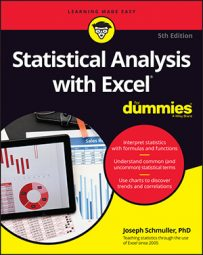##### Statistical Analysis with Excel For DummiesSpearman’s correlation coefficient, rS, was the earliest non-parametric test based on ranks. For a sample of individuals each measured on two variables in Excel , the idea is to rank each score within its own variable. Then, for each individual subtract one rank from the other. If correlation is perfect (in the positive direction), all the differences are zero.

The image below shows an example. An industrial psychologist rated the sociability of 20 employees of the FarDrate Timepiece Corporation. The scale ranged from 1 (least sociable) to 100 (most sociable). Each FarDrate employee also rated his or her job satisfaction on a scale of 1 (least satisfaction) to 80 (most satisfaction). The null hypothesis is that sociability is not correlated with job satisfaction. The alternative hypothesis is that these two variables are correlated.

The data are in columns B and C, and the ranks are in columns E and F. The differences between each pair of ranks are in column G.

The formula is

where d is an interpair difference. As is the case with the regular correlation coefficient, if the null hypothesis is true, the value of rS should be around zero.

To calculate the ranks in column E, type

`=RANK.AVG(B2,\$B\$2:\$B\$21,1)`

into E2 and autofilled. For the ranks in column E, type

`=RANK.AVG(C2,\$C\$2:\$C\$21,1)`

into F2 and autofilled.

You don’t have to type a complicated Excel formula into cell J4 to calculate the correlation coefficient. Why? Because Excel and mathematical statistics team up for a swell surprise: All you have to do is type

`=CORREL(E2:E21,F2:F21)`

into J4. That’s all there is to it. Using `CORREL` on the ranks gives the same answer as the formula above. (So it isn’t really necessary to calculate the interpair rank differences in column G.)

Calculate

N is the number of pairs, and the test has N-2 degrees of freedom.

You can define `Number_of_pairs` as the name for the value in cell I2. So type

`=J4*SQRT(Number_of_Pairs-2)/SQRT(1-J4^2)`

into J6 and

`=T.DIST.2T(J6,Number_of_Pairs-2)`

into J7. You can use the two-tailed t distribution function if you don’t know the correlation’s direction in advance. And once again, the low p-value tells you to reject the null hypothesis.